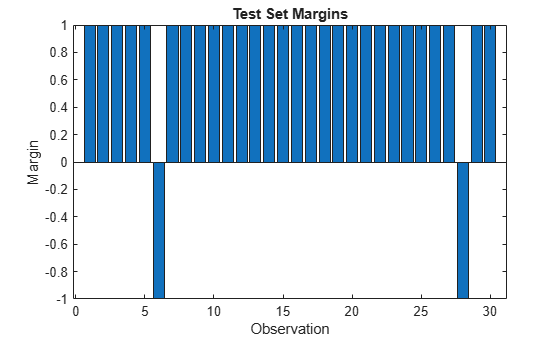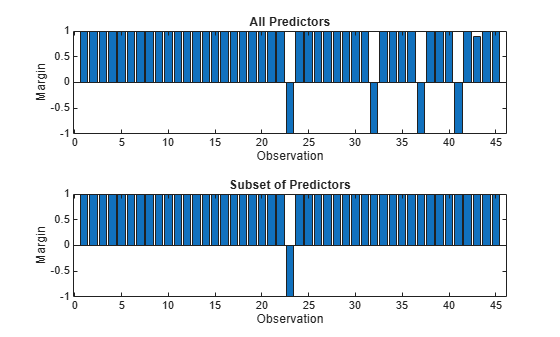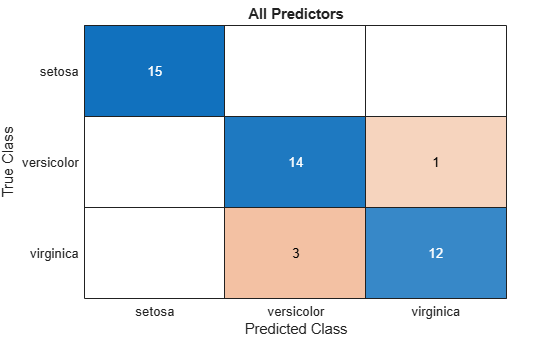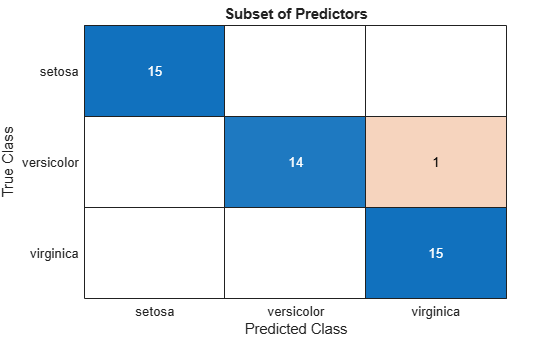# margin

Classification margins for neural network classifier

## Syntax

``m = margin(Mdl,Tbl,ResponseVarName)``
``m = margin(Mdl,Tbl,Y)``
``m = margin(Mdl,X,Y)``
``m = margin(Mdl,X,Y,'ObservationsIn',dimension)``

## Description

example

````m = margin(Mdl,Tbl,ResponseVarName)` returns the classification margins for the trained neural network classifier `Mdl` using the predictor data in table `Tbl` and the class labels in the `ResponseVarName` table variable.`m` is returned as a numeric vector, whose ith entry corresponds to the ith observation in `Tbl`.```
````m = margin(Mdl,Tbl,Y)` returns the classification margins for the classifier `Mdl` using the predictor data in table `Tbl` and the class labels in vector `Y`.```
````m = margin(Mdl,X,Y)` returns the classification margins for the trained neural network classifier `Mdl` using the predictor data `X` and the corresponding class labels in `Y`.`m` is returned as a numeric vector, whose ith entry corresponds to the ith observation in `X`.```
````m = margin(Mdl,X,Y,'ObservationsIn',dimension)` specifies the predictor data observation dimension, either `'rows'` (default) or `'column'`. For example, specify `'ObservationsIn','columns'` to indicate that columns in the predictor data correspond to observations.```

## Examples

collapse all

Calculate the test set classification margins of a neural network classifier.

Load the `patients` data set. Create a table from the data set. Each row corresponds to one patient, and each column corresponds to a diagnostic variable. Use the `Smoker` variable as the response variable, and the rest of the variables as predictors.

```load patients tbl = table(Diastolic,Systolic,Gender,Height,Weight,Age,Smoker);```

Separate the data into a training set `tblTrain` and a test set `tblTest` by using a stratified holdout partition. The software reserves approximately 30% of the observations for the test data set and uses the rest of the observations for the training data set.

```rng("default") % For reproducibility of the partition c = cvpartition(tbl.Smoker,"Holdout",0.30); trainingIndices = training(c); testIndices = test(c); tblTrain = tbl(trainingIndices,:); tblTest = tbl(testIndices,:);```

Train a neural network classifier using the training set. Specify the `Smoker` column of `tblTrain` as the response variable. Specify to standardize the numeric predictors.

```Mdl = fitcnet(tblTrain,"Smoker", ... "Standardize",true);```

Calculate the test set classification margins. Because the test set includes only 30 observations, display the margins using a bar graph.

```m = margin(Mdl,tblTest,"Smoker"); bar(m) xlabel("Observation") ylabel("Margin") title("Test Set Margins")```Only the sixth and twenty-eighth observations have negative margins, which indicates that the model performs well overall.

Perform feature selection by comparing test set classification margins, edges, errors, and predictions. Compare the test set metrics for a model trained using all the predictors to the test set metrics for a model trained using only a subset of the predictors.

Load the sample file `fisheriris.csv`, which contains iris data including sepal length, sepal width, petal length, petal width, and species type. Read the file into a table.

`fishertable = readtable('fisheriris.csv');`

Separate the data into a training set `trainTbl` and a test set `testTbl` by using a stratified holdout partition. The software reserves approximately 30% of the observations for the test data set and uses the rest of the observations for the training data set.

```rng("default") c = cvpartition(fishertable.Species,"Holdout",0.3); trainTbl = fishertable(training(c),:); testTbl = fishertable(test(c),:);```

Train one neural network classifier using all the predictors in the training set, and train another classifier using all the predictors except `PetalWidth`. For both models, specify `Species` as the response variable, and standardize the predictors.

```allMdl = fitcnet(trainTbl,"Species","Standardize",true); subsetMdl = fitcnet(trainTbl,"Species ~ SepalLength + SepalWidth + PetalLength", ... "Standardize",true);```

Calculate the test set classification margins for the two models. Because the test set includes only 45 observations, display the margins using bar graphs.

For each observation, the classification margin is the difference between the classification score for the true class and the maximal score for the false classes. Because neural network classifiers return classification scores that are posterior probabilities, margin values close to 1 indicate confident classifications and negative margin values indicate misclassifications.

```tiledlayout(2,1) % Top axes ax1 = nexttile; allMargins = margin(allMdl,testTbl); bar(ax1,allMargins) xlabel(ax1,"Observation") ylabel(ax1,"Margin") title(ax1,"All Predictors") % Bottom axes ax2 = nexttile; subsetMargins = margin(subsetMdl,testTbl); bar(ax2,subsetMargins) xlabel(ax2,"Observation") ylabel(ax2,"Margin") title(ax2,"Subset of Predictors")```Compare the test set classification edge, or mean of the classification margins, of the two models.

`allEdge = edge(allMdl,testTbl)`
```allEdge = 0.8198 ```
`subsetEdge = edge(subsetMdl,testTbl)`
```subsetEdge = 0.9556 ```

Based on the test set classification margins and edges, the model trained on a subset of the predictors seems to outperform the model trained on all the predictors.

Compare the test set classification error of the two models.

```allError = loss(allMdl,testTbl); allAccuracy = 1-allError```
```allAccuracy = 0.9111 ```
```subsetError = loss(subsetMdl,testTbl); subsetAccuracy = 1-subsetError```
```subsetAccuracy = 0.9778 ```

Again, the model trained using only a subset of the predictors seems to perform better than the model trained using all the predictors.

Visualize the test set classification results using confusion matrices.

```allLabels = predict(allMdl,testTbl); figure confusionchart(testTbl.Species,allLabels) title("All Predictors")``````subsetLabels = predict(subsetMdl,testTbl); figure confusionchart(testTbl.Species,subsetLabels) title("Subset of Predictors")```The model trained using all the predictors misclassifies four of the test set observations. The model trained using a subset of the predictors misclassifies only one of the test set observations.

Given the test set performance of the two models, consider using the model trained using all the predictors except `PetalWidth`.

## Input Arguments

collapse all

Trained neural network classifier, specified as a `ClassificationNeuralNetwork` model object or `CompactClassificationNeuralNetwork` model object returned by `fitcnet` or `compact`, respectively.

Sample data, specified as a table. Each row of `Tbl` corresponds to one observation, and each column corresponds to one predictor variable. Optionally, `Tbl` can contain an additional column for the response variable. `Tbl` must contain all of the predictors used to train `Mdl`. Multicolumn variables and cell arrays other than cell arrays of character vectors are not allowed.

• If `Tbl` contains the response variable used to train `Mdl`, then you do not need to specify `ResponseVarName` or `Y`.

• If you trained `Mdl` using sample data contained in a table, then the input data for `margin` must also be in a table.

• If you set `'Standardize',true` in `fitcnet` when training `Mdl`, then the software standardizes the numeric columns of the predictor data using the corresponding means and standard deviations.

Data Types: `table`

Response variable name, specified as the name of a variable in `Tbl`. If `Tbl` contains the response variable used to train `Mdl`, then you do not need to specify `ResponseVarName`.

If you specify `ResponseVarName`, then you must specify it as a character vector or string scalar. For example, if the response variable is stored as `Tbl.Y`, then specify `ResponseVarName` as `'Y'`. Otherwise, the software treats all columns of `Tbl`, including `Tbl.Y`, as predictors.

The response variable must be a categorical, character, or string array; a logical or numeric vector; or a cell array of character vectors. If the response variable is a character array, then each element must correspond to one row of the array.

Data Types: `char` | `string`

Class labels, specified as a categorical, character, or string array; logical or numeric vector; or cell array of character vectors.

• The data type of `Y` must be the same as the data type of `Mdl.ClassNames`. (The software treats string arrays as cell arrays of character vectors.)

• The distinct classes in `Y` must be a subset of `Mdl.ClassNames`.

• If `Y` is a character array, then each element must correspond to one row of the array.

• The length of `Y` must be equal to the number of observations in `X` or `Tbl`.

Data Types: `categorical` | `char` | `string` | `logical` | `single` | `double` | `cell`

Predictor data, specified as a numeric matrix. By default, `margin` assumes that each row of `X` corresponds to one observation, and each column corresponds to one predictor variable.

Note

If you orient your predictor matrix so that observations correspond to columns and specify `'ObservationsIn','columns'`, then you might experience a significant reduction in computation time.

The length of `Y` and the number of observations in `X` must be equal.

If you set `'Standardize',true` in `fitcnet` when training `Mdl`, then the software standardizes the numeric columns of the predictor data using the corresponding means and standard deviations.

Data Types: `single` | `double`

Predictor data observation dimension, specified as `'rows'` or `'columns'`.

Note

If you orient your predictor matrix so that observations correspond to columns and specify `'ObservationsIn','columns'`, then you might experience a significant reduction in computation time. You cannot specify `'ObservationsIn','columns'` for predictor data in a table.

Data Types: `char` | `string`

collapse all

### Classification Edge

The classification edge is the mean of the classification margins.

One way to choose among multiple classifiers, for example, to perform feature selection, is to choose the classifier that yields the greatest edge.

### Classification Margin

The classification margin for binary classification is, for each observation, the difference between the classification score for the true class and the classification score for the false class. The classification margin for multiclass classification is the difference between the classification score for the true class and the maximal score for the false classes.

If the margins are on the same scale (that is, the score values are based on the same score transformation), then they serve as a classification confidence measure. Among multiple classifiers, those that yield greater margins are better.

## Version History

Introduced in R2021a